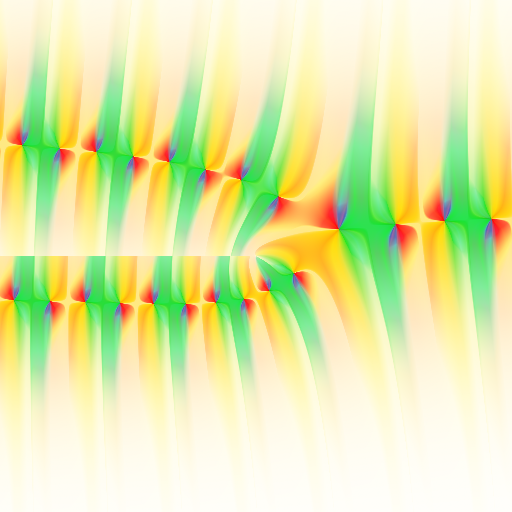# Arb 2.17 released

October 16, 2019

I have just tagged version 2.17.0 of Arb. As usual, downloads are available on GitHub, and there is a changelog in the documentation. The feature list is relatively short, in part because I've spent a lot of time working on the Mathematical Functions Grimoire the last several months. In any case, there are a couple of highlights worth mentioning.

## Riemann zeta function zeros

Contributed by the mysterious D.H.J. Polymath, Arb now has code for computing zeros of the Riemann zeta function with given index, as well as counting the number of zeros in the critical strip up to a given height. Code example using the development version of Python-FLINT:

>>> from flint import *
>>> arb(10**10).zeta_nzeros()
32130158315.0000

>>> acb.zeta_zero(10**10)
0.500000000000000 + [3293531632.39714 +/- 3.80e-6]j
>>> ctx.dps = 30
>>> acb.zeta_zero(10**10)
0.500000000000000000000000000000 + [3293531632.39713670420899170313 +/- 3.91e-21]j


The functionality is similar to the zetazero and nzeros functions originally implemented in mpmath by Juan Arias de Reyna. The new Arb implementations are loosely based on the algorithms in mpmath (a version of Turing's method), but have some improvements for performance, and thanks to using ball arithmetic they should also give certified correct results.

To give a quick idea of the difference in speed, Arb computes the first 1000 nontrivial zeros to 15 digits in 3.7 seconds, while mpmath takes 5 minutes; almost 100 times faster. Arb computes the $n = 10^{10}$ zero to 30 digits in 0.3 seconds while mpmath takes 2.3 seconds.

There is also code for computing many zeros simultaneously which should be even faster, but I have not experimented much with it yet.

## Serialization

Contributed by Julian Rüth, there are now functions to dump the exact content of an arb_t to a string or a file and read it back again. This avoids the slight inflation of the ball resulting from a binary–decimal–binary conversion roundtrip with the normal printing functions, and should be much faster too. It's a simple but important feature that should have been in the library much earlier!

## Coulomb wave functions

Arb now implements the Coulomb wave functions $F_{\ell}(\eta,z), G_{\ell}(\eta,z), H^{+}_{\ell}(\eta,z), H^{-}_{\ell}(\eta,z)$ as well as their derivatives, for real and complex variables. The Coulomb wave functions are just special cases of confluent hypergeometric functions, but the normalization factors and branch cuts are a bit complicated to deal with, so it's convenient to have them directly in the library.Plot of $G_{1,2+i}(z)$ on $z \in [-20,20] + [-20,20] i$.